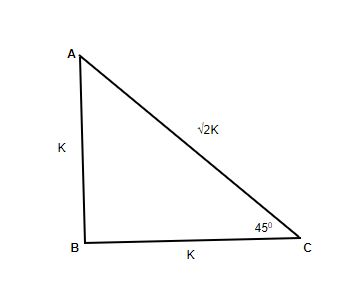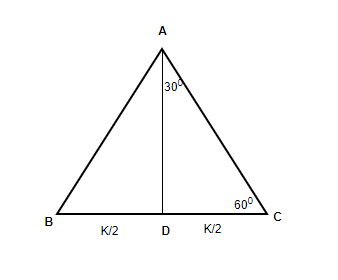How to evaluate trigonometric functions without a calculator?

• Last Updated : 28 Sep, 2021

Trigonometry is known as the branch of mathematics that deals with certain measurements of triangular regions. A common application of trigonometry is the measurement of the sides and angles of a triangle. For this, we use some trigonometric functions of acute angles. These functions are defined as certain ratios of a right-angled triangle containing the angle. The values of trigonometric ratios of certain angles, called standard angles, can be obtained geometrically. These angles are 0°, 30° or π/6, 45° or π/4, 60° or π/3 and 90° or π/2.

Trigonometric Identities

In mathematics, trigonometric identities are equalities that involve trigonometric functions and are true for each value of the occurring variables that each side of the equality is defined.

Co-function Identities

• sin θ = cos(90° – θ)
• sec θ = cosec(90° – θ)
• tan θ = cot(90° – θ)

Supplement Angle Identities

• sin(π – θ) = sin θ.
• cos(π – θ) = -cos θ
• tan(π – θ) = – tan θ
• cosec(π – θ) = cosec θ
• sec(π – θ) = -sec θ
• cot(π – θ) = -cot θ

Values of Trigonometric Ratios of 45°.

Let ABC be a right-angled isosceles triangle in which ∠ABC = 90° and AB = BC. From geometry, ∠ABC = ∠BAC = 45°.If BC = k then AB = k.

∴ AB2 + BC2 = AC2 (By Pythagoras Theorem)

∴ k2 + k2 = AC2, or  AC2 =2k2.

∴ AC = √2 k.

Now,  sin 45° = sin C= p/h = AB/AC = k/√2 k = 1/√2

cos 45° = cos C = b/h = BC/AC = k/√2 k = 1/√2

tan 45°= tan C= p/b = AB/BC = k/ k = 1

cosec, sec, cot being the reciprocal of sin, cos, tan respectively will have just the reciprocal values as follows cosec 45°= √2 ,

sec 45° = √2  and cot 45° = 1.

Values of Trigonometric Ratios of 30° and 60°

Let ABC be an equilateral triangle whose each side is k. By geometry, each angle of the triangle = 60°. Let AD⊥BC. From geometry, AD bisects ∠BAC and it also bisects the side BC.∴ ∠CAD = ∠BAD = 30° and CD = BD = k/2.

AD + k2/2 = k2 OR AD = k2 – k2/4 = 3k2/4.

sin 30° = sin∠CAD = p/h = CD/AC = (k/2)/k = 1/2

cos 30° = cos∠CAD = b/h = AD/AC = (√3k/2)/k = √3/2

tan 30° = tan∠CAD = p/b = CD/AD = (k/2)/(√3k/2) = 1/√3

As shown earlier, cosec 30°, sec 30°, cot 30° being the reciprocal of sin, cos, tan respectively will have just the reciprocal values.

sin 60° = sin∠CAD = p/h = AD/AC = (√3k/2)/k = √3/2

cos 60° = cos∠CAD = b/h = CD/AC = (k/2)/k =1/2

tan 60° = tan∠CAD = p/b = AD/CD = (√3k/2)/(k/2) = √3

Also, cosec 60°, sec 60°, cot 60° being the reciprocal of sin, cos, tan respectively will have just the reciprocal values.

Values of Trigonometric Ratios of 0° and 90°

In a right-angled triangle the measure of no angle can be 0°, and neither can there be another angle of 90°. As we have seen, the triangle ratios of θ (when 0° < θ < 90°) can be obtained from their definitions. The values of trigonometric ratios turn out as follows.

sin 90°= 0, cos 0° = 1, tan 0° = 0, sec 0° = 1,

sin 90° = 1, cos 90° = 0, cot 90° = 0, sec 90° = 1.

Other trigonometric ratios of 0° and 90° are not defined.

Tabulated values

How to evaluate trigonometry functions without a calculator?

As known, there are four quadrants in trigonometry, the first quadrant being all positive trigonometric values, the second quadrant is where only sine and cosec are positive, in the third quadrant only tan and cot are positive, and in the fourth one cosine and sec are positive. (Go Anti-clockwise from Right-hand Top).

The above given trigonometric ratios of standard values, as well as the trigonometric identities, will help us to find an angle in trigonometry without a calculator. If sin 150° is given to find out, we can write or elaborate this as,

Steps

1. Simplify sin 150° into sin(90° + 60°).
2. Further, we can say sin((1 × 90°) + 60°)
3. Now as we have an odd coefficient of 90°, sin changes to cos.
4. Also, it is covering 90° in the first quadrant and again added 60°  leads to the second quadrant. In the second quadrant, we know sine is positive.
5. The final result will be cos 60°.

Note The standard values should be memorized.

Sample Problems

Question 1: Find tan 135° without using a calculator.

Solution:

tan 135° = tan(90° + 45°) = tan((1 × 90°) + 45°) = -cot 45° = -1.

Explanation As here too, an odd coefficient of 90° is present, so tan changes to the cot, and also it’s coming to be in the second quadrant where only sine and cosine are positive and rest all are negative. Hence the result of tan 135° = – cot 45° = -1.

Remember If the coefficient of 90° is odd, sine changes to cos, tan changes to cot, sec changes to cosec. If the coefficient of 90° is even, the function remains as it is and depending on the quadrant, the sign (+ or -) will appear.

Another way to solve, knowing the formulas of addition and subtraction identities such as sin(x + y) = sin(x)cos(y) + cos(x)sin(y)  and cos(x + y) = cos(x) cos (y) – sin(x) sin(y).

Question 2: Find cos 330°.

Solution:

cos(270° + 60°) = (cos 270° × cos 60°) – (sin 270°× sin 60°) = {cos(90° × 3) × 1/2} – {sin(90° × 3) × √3/2}

= (-sin 0° × 1/2 ) – (-cos 0°× √3/2) = 0 + √3/2 = √3/2 = 0.866

Question 3: Find sec 120°.

Solution:

sec 120° = sec(90° + 30°) = -cosec 30° = -2.

Explanation Here, an odd coefficient of 90° is present, so sec changes to cosec, and also it’s coming to be in the second quadrant where only sine and cosec are positive and rest all are negative. Hence, -cosec 30° is obtained.

Question 4: Find sin 390°.

Solution:

sin 390° = sin(4 × 90° + 30°) = -sin 30° = -1/2 = -0.5 .

Explanation Here, even a coefficient of 90° is present, so sin remains as it is and it’s coming to be in the fourth quadrant where only sec and cos are positive and the rest all are negative. Hence, we get -sin 30°.

Question 5: Find cot 150°.

Solution:

cot 150° = cot(2 × 90° – 30°) = -cot 30° = -√3.

Explanation Here, even a coefficient of 90° is present, so cot remains as it is and it’s coming to be in the second quadrant where only sin and cosec are positive and the rest all are negative. Hence, we get -cot 30°.

My Personal Notes arrow_drop_up